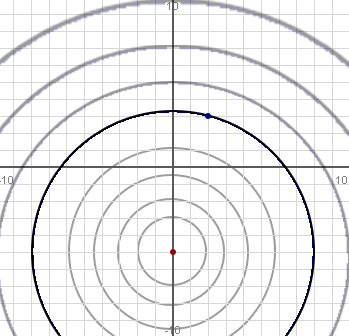SEARCH HOMEMath Central Quandaries & QueriesQuestion from Raymund, a student: Find the radius if the center is at (0, -5) and one point on the circle is (2,3) Thanks.Hi Raymund.

The standard form of the equation of a circle is

(x - x0)2 + (y - y0)2 = r2

where (x0, y0) is the center of the circle
and r is its radius.

So you can substitute in (0, -5) for the center (x0, y0) and you will get an equation involving x, y and r.

You are told that the point (2, 3) is on the circle, which means it "solves" the equation. So now substitute (2, 3) in for (x, y) in your equation and you will be able to find the radius r.

Cheers,
Stephen La Rocque.Math Central is supported by the University of Regina and The Pacific Institute for the Mathematical Sciences.